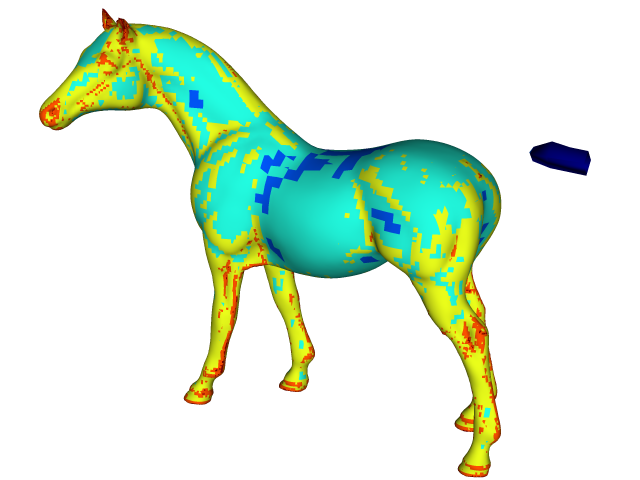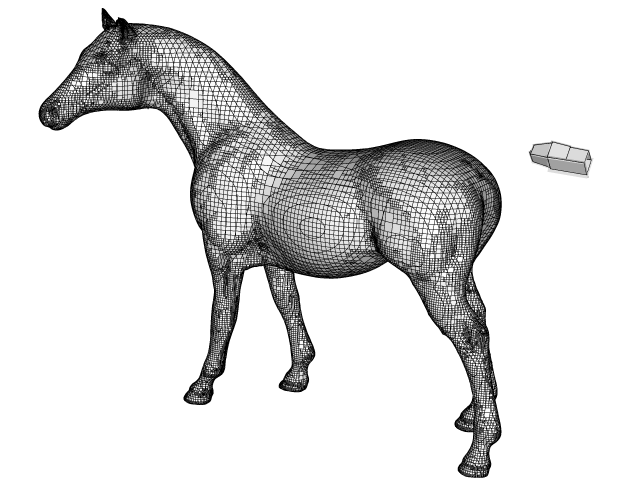# Distance field computation from a 3D model

The goal is to build a distance field representation of a 3D CAD model.

#include "grid/octree.h"
#include "utils.h"
#include "distance.h"
#include "fractions.h"
#include "view.h"

int main()
{

We get the 3D model from the Large Geometric Models Archive. This is in PLY format which we need to convert to STL using meshlab.

  system ("test -f distance.stl || "
"(wget http://www.cc.gatech.edu/data_files/large_models/horse.ply.gz && "
"gunzip -f horse.ply.gz && "
"meshlabserver -i horse.ply -o distance.stl)");

We read the STL file, compute the bounding box of the model and set the domain center and size using this bounding box.

  coord * p = input_stl (fopen ("distance.stl", "r"));
coord min, max;
bounding_box (p, &min, &max);
double maxl = -HUGE;
foreach_dimension()
if (max.x - min.x > maxl)
maxl = max.x - min.x;

init_grid (8);
size (1.2*maxl);
origin ((max.x + min.x)/2. - L0/2,
(max.y + min.y)/2. - L0/2,
(max.z + min.z)/2. - L0/2);

We initialize the distance field on the coarse initial mesh and refine it adaptively until the threshold error (on distance) is reached.

  scalar d[];
distance (d, p);
while (adapt_wavelet ({d}, (double[]){5e-4*L0}, 10).nf);

We display an isosurface of the distance function coloured with the level of refinement.

  view (fov = 15.65, quat = {-0.52,0.31,0.38,-0.7},
tx = -0.045, ty = 0.015, width = 640, height = 480, bg = {1,1,1});
isosurface ("d", 0, color = "level", min = 5, max = 10);
save ("horse.png");

We also compute the volume and surface fractions from the distance field.

  scalar f[];
face vector s[];
solid (f, s, (d[] + d[-1] + d[0,-1] + d[-1,-1] +
d[0,0,-1] + d[-1,0,-1] + d[0,-1,-1] + d[-1,-1,-1])/8.);

Finally we display the surface reconstructed from volume fractions.

  clear();
draw_vof ("f", "s");
draw_vof ("f", "s", edges = true, lw = 0.5);
save ("vof.png");
}

Note that the “tail” of the horse is an artefact due to an inconsistency in the surface mesh, which is self-intersecting near this point.Isosurface of the distance function coloured with level of refinement.Reconstructed VOF surface.